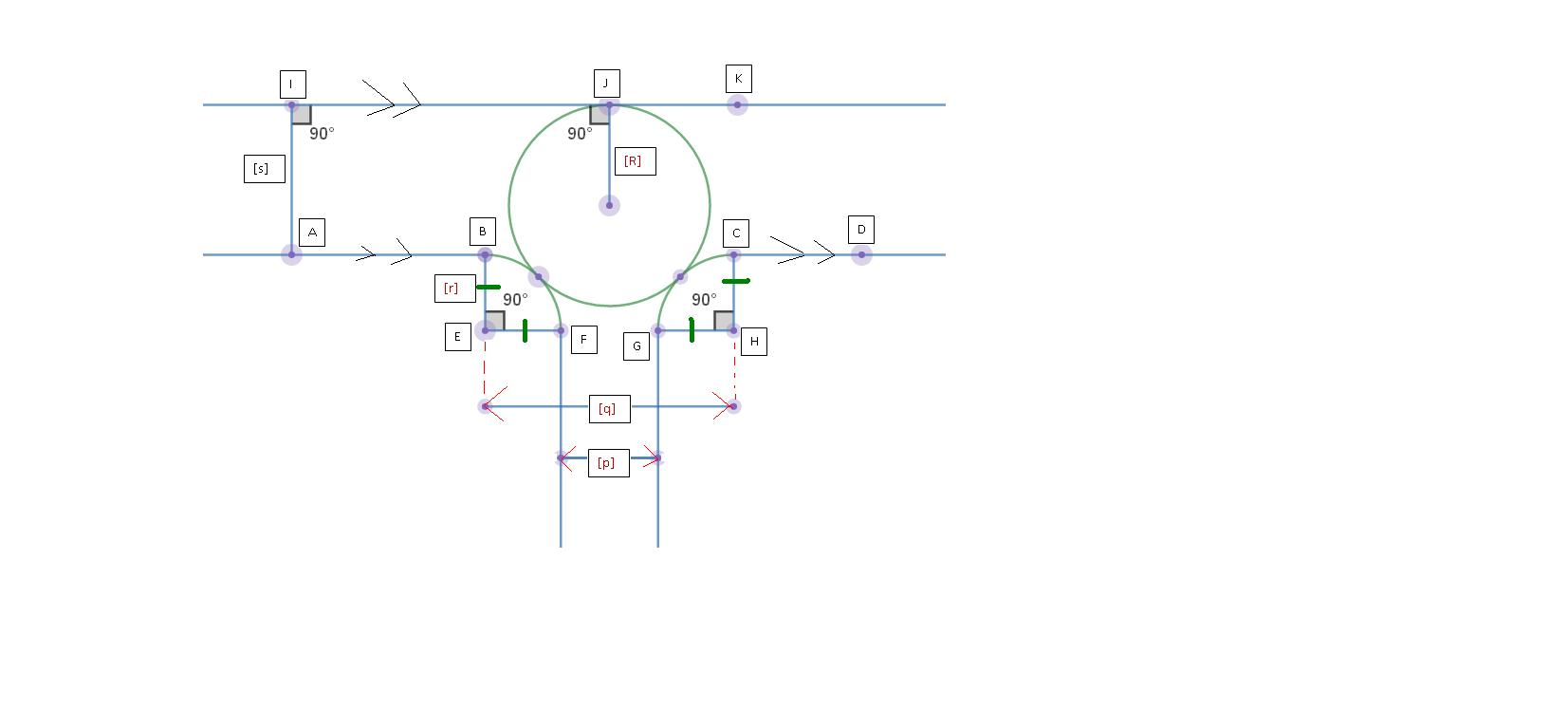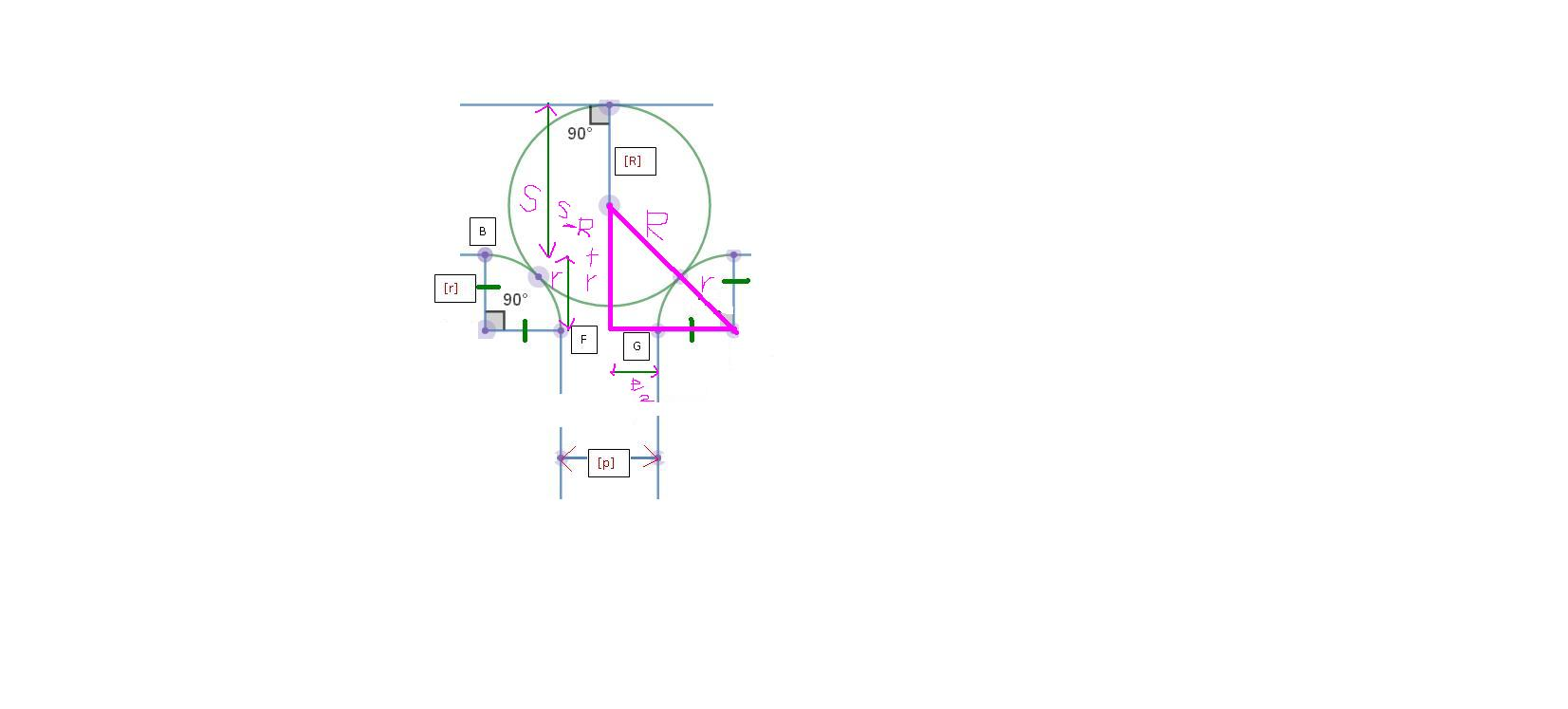# Tough Geometry I!---Just for personal reference

Diagram shows a straight line $\text{IJK}$, a circle, $2$ congruent quadrants $\text{BEF}$ and $\text{CGH}$, and straight lines extended from the ends of each arc. The top centre circle is tangent to the upper line at $\text{J}$ and the two quadrants at the marked points.

$\text{AB}$ and $\text{CD}$ are collinear

Given:

$p = \text{Distance of FG}$

$q = \text{Distance of BC} = \text{Distance if EH}$

$r = \text{Radius of each quadrant}$

$s = \text{Perpendicular distance from IJK to AB or CD}$

$R = \text{ Radius of the circle}$

The relation between $p, q, r, s, \text{and} R$:

$(s + r - R)^2 + (\dfrac{p}{2} + r)^2 = (R + r)^2$

Using Pythagorean Theorem

Note :The figure is symmetrical about the vertical diameter line of the circle (ignoring extra lines and points)Note by Lin Shun Hao
1 year, 1 month ago

This discussion board is a place to discuss our Daily Challenges and the math and science related to those challenges. Explanations are more than just a solution — they should explain the steps and thinking strategies that you used to obtain the solution. Comments should further the discussion of math and science.

When posting on Brilliant:

• Use the emojis to react to an explanation, whether you're congratulating a job well done , or just really confused .
• Ask specific questions about the challenge or the steps in somebody's explanation. Well-posed questions can add a lot to the discussion, but posting "I don't understand!" doesn't help anyone.
• Try to contribute something new to the discussion, whether it is an extension, generalization or other idea related to the challenge.
• Stay on topic — we're all here to learn more about math and science, not to hear about your favorite get-rich-quick scheme or current world events.

MarkdownAppears as
*italics* or _italics_ italics
**bold** or __bold__ bold
- bulleted- list
• bulleted
• list
1. numbered2. list
1. numbered
2. list
Note: you must add a full line of space before and after lists for them to show up correctly
paragraph 1paragraph 2

paragraph 1

paragraph 2

[example link](https://brilliant.org)example link
> This is a quote
This is a quote
    # I indented these lines
# 4 spaces, and now they show
# up as a code block.

print "hello world"
# I indented these lines
# 4 spaces, and now they show
# up as a code block.

print "hello world"
MathAppears as
Remember to wrap math in $$ ... $$ or $ ... $ to ensure proper formatting.
2 \times 3 $2 \times 3$
2^{34} $2^{34}$
a_{i-1} $a_{i-1}$
\frac{2}{3} $\frac{2}{3}$
\sqrt{2} $\sqrt{2}$
\sum_{i=1}^3 $\sum_{i=1}^3$
\sin \theta $\sin \theta$
\boxed{123} $\boxed{123}$

## Comments

There are no comments in this discussion.

×

Problem Loading...

Note Loading...

Set Loading...